# Pattern creations by sensing body motion 2

BEND SENSORS

Connecting 3 bend sensors with 3 resistors (voltage divider) to 3 analog pins A0, A1 and A2 on the Arduino board. Bend sensor change the value when it’s bended.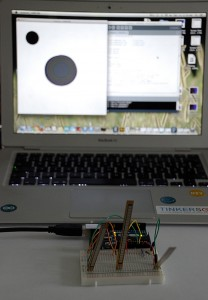I was reading the values of the bend sensors (with AnalogRedSerial) through Serial monitor and noticed a small range: from 140 as minimum value to 390 maximum.

Drawing with bend sensors

I coded different Processing drawing application using dots:

v15

v14

draw2

Dots are drawing the colour changing line and square. Because of the small range. I didn’t mapped the min and max value. Later I used the same code with textile sensors and output was different.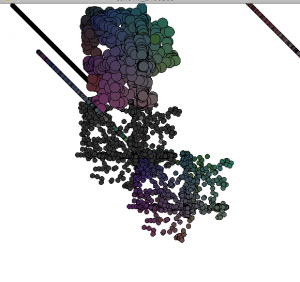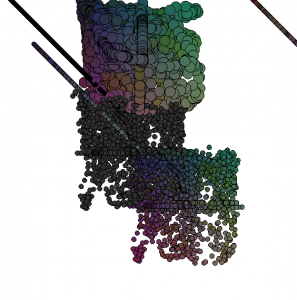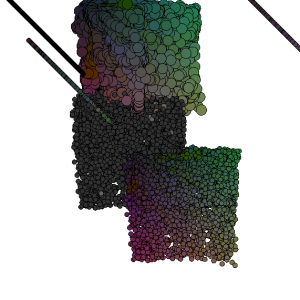And Processing code for the last video:

import processing.serial.*;

float redValue = 0;
float greenValue = 0;
float blueValue = 0;

float mPos=0;
float nPos=0;
float oPos=0;

Serial myPort;

void setup() {
size(600, 600);
background(255);

println(Serial.list());
myPort = new Serial(this, Serial.list(), 9600);
myPort.bufferUntil(‘\n’);
}

void draw() {

fill (100,100,100);

ellipse (mPos+100,nPos+100, 9,9);

fill (greenValue, redValue, blueValue);
ellipse (mPos+200,nPos+200, 9, 9);

fill (50,50,50);
ellipse (mPos+100,nPos+100, 9,9);

fill (blueValue, redValue, greenValue);
ellipse (nPos+400,nPos-100, 9,9);

fill (0);
ellipse (nPos-40,oPos-80, 9,9);

fill (redValue, greenValue, blueValue);
ellipse (oPos+100,mPos-60, 20,20);

fill (redValue, greenValue, blueValue);
ellipse (nPos+10,nPos+10, 9,9);
}

void serialEvent(Serial myPort) {
if (inString != null) {
inString = trim(inString);
float[] colors = float(split(inString, “,”));
if (colors.length >=3) {
redValue = map(colors, 0, 1023, 0, 255);
greenValue = map(colors, 0, 1023, 0, 255);
blueValue = map(colors, 0, 1023, 0, 255);

mPos=map(colors, 0, 1023, 0, width);
nPos=map(colors, 0, 1023, 0, height);
oPos=map(colors, 0, 1023, 0, width);
}
}
}

I realised if I put background settings in void setup the elements are adding and drawing patterns and if I put it to void draw I can move elements around the screen.

dwar_move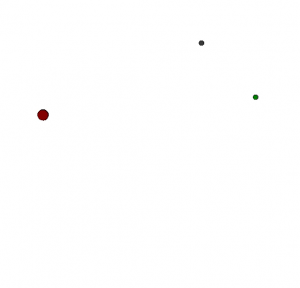The code is the same just change in background setting.